hopfion.com
Hopf solitons in modern mathematical physics

# Bose-Einstein condensate

## The model description

Bose-Einstein condensate is described by the Gross-Pitaevskii equation. Consider the Hamiltonian density, which was proposed in [T.L. Ho "Spinor Bose Condensates in Optical Traps", arXiv:cond-mat/9803231v1; Phys. Rev. Lett. 81, 742 (1998) ref]: (1.1)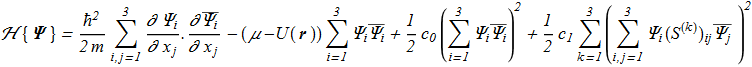where: (1.2)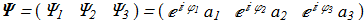is a vector of three complex variables and spin-1 matrices (1.3)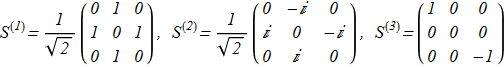Euler-Lagrange equations: (1.4)(1.5)The solution of equations corresponding to the so-called "polar" phase: (1.6)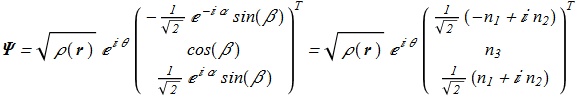(1.7)with equation on amplitude (1.8)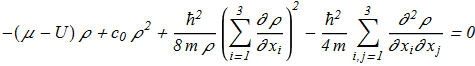But also the solution exists where the n-vector field is not homogeneous in space: (1.9)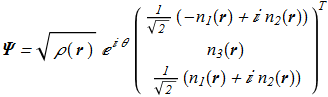(1.10)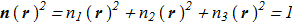with 3 partial differential equations from {1.4}-{1.5} for ρ and two n-vector parametrizing angles. The corresponding Hamiltonian density: (1.11)If we introduce the definition (1.12)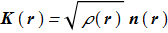we get (1.13)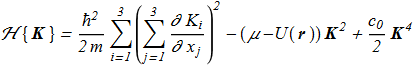## Knot or not ?

The appearance of a unit vector field in the Hamiltonian leads to some speculations [Y. Kawaguchi, M. Nitta, M. Ueda "Knots in a Spinor Bose-Einstein Condensate", arXiv:0802.1968v1; Phys. Rev. Lett. 100, 180403 (2008) ref], [Y. Kawaguchi, M. Kobayashi, M. Nitta, M. Ueda "Topological Excitations in Spinor Bose-Einstein Condensates", arXiv:1006.5839v1; Prog. Theor. Phys. Suppl. 186 (2010) 455, ref] about the existence of knots with nonzero Hopf index. However, the presence of the scalar density in {1.11} can not be simply discarded. The constant value ρ=const does not correspond to an extremum of the Hamiltonian {1.11} with non-homogeneous n-field. Instead of map (2.1)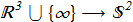we have a map for {1.13} (2.2)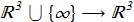which is homotopic to zero. Any configuration of the n-field can be cutted into regions with zero vector amplitude (ρ=0), and each knot for n-field can be destroyed, while the original function {1.2} can be continuous. This fact means that even if the parametric space are include some Hopf fibration, it is not topologically stable knot, it is not a hopfion in the strict mathematical sense.

Similar problems arise for other representations of the energy functionals, such as [E. Babaev, L.D. Faddeev, A.J. Niemi "Hidden symmetry and knot solitons in a charged two-condensate Bose system", arXiv:cond-mat/0106152v1; Phys. Rev. B 65 100512 (2002) ref].